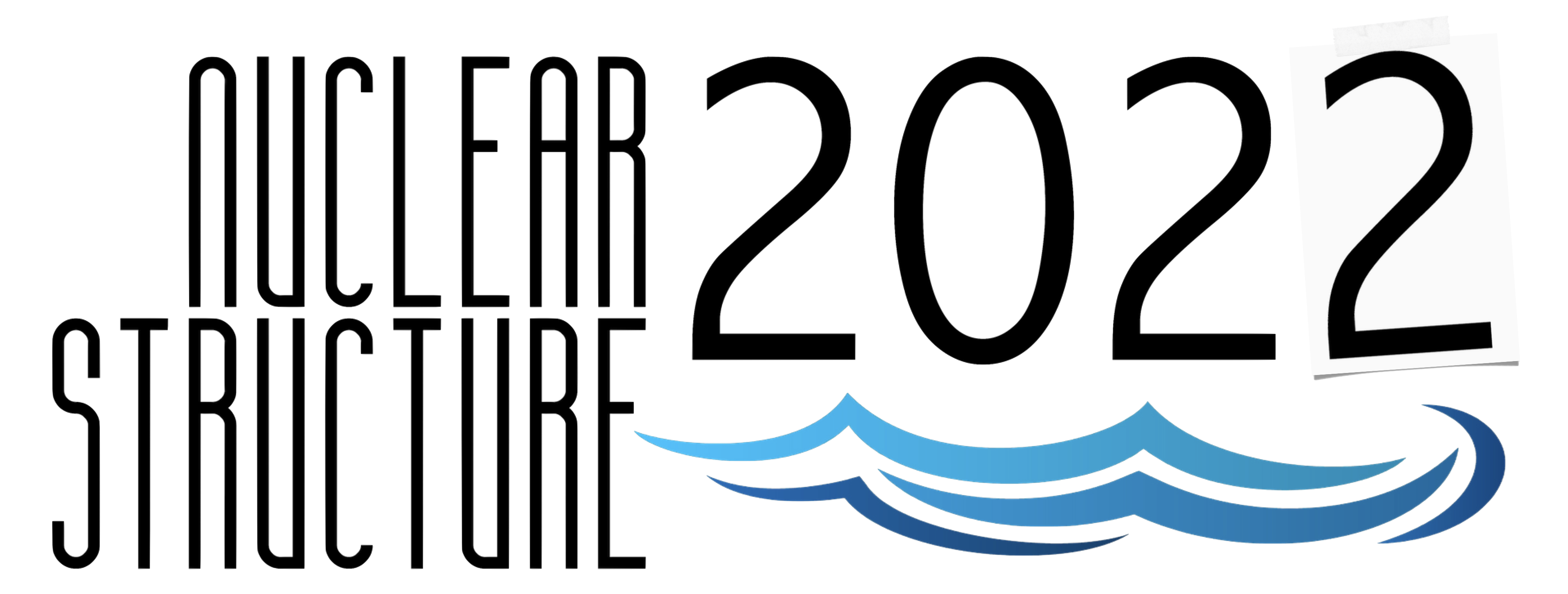#Nuclear Structure 2022

Jun 13 – 17, 2022
Berkeley, CA
US/Pacific timezone

## Beta decay strength of $^{78}$Ni to neutron-unbound states revealed by $^{79}$Cu

Jun 16, 2022, 4:10 PM
20m
Berkeley, CA

#### Berkeley, CA

Lawrence Berkeley National Laboratory
Oral Oral Presentations

### Speaker

Maninder Singh (University of Tennessee, Knoxville)

### Description

$^{78}$Ni is one of the few radioactive doubly-magic nuclei on the nuclear landscape . It has a large neutron-to-proton ratio and engages in beta-delayed neutron and gamma emissions. Decays of Cu isotopes with significant neutron excess provide a laboratory to study such doubly-magic nuclei. The nuclear shell structure of $^{79}$Cu (N=50; Z=29) is highly similar to the one of $^{78}$Ni (N=50; Z=28), except an extra proton above the Z=28 shell gap in $1f_{5/2}$ orbital . The similarities in the nuclear shell structure are also reflected in the beta decay properties. $^{79}$Cu is also a strong beta-delayed neutron emitter, which allows us to infer beta-strength function $(S_{\beta}(E))$ to neutron-unbound states in the beta decay of $^{78}$Ni.

The determination of $(S_{\beta}(E))$ requires measurement of the intensity of neutron resonances post beta decay. Neutron-gamma coincidences are also vital to arranging strength with excitation energy. An experiment for measuring the strength distribution in the $^{78}$Ni region (27$\geq$Z$\geq$33) was performed at the Radioactive Ion Beam Factory (RIBF) facility at RIKEN Nishina Center, JAPAN using a YSO-based implantation detector  and VANDLE  array for Time-of-Flight-based spectroscopy of the beta-delayed neutrons. For $\gamma$-ray detection, two HPGe clovers and 10 $LaBr_{3}$ detectors were used.

This work reports the first direct measurements of beta-decay strength to neutron-unbound states in the decay of $^{81, 80, 79}$Cu crossing over the N=50 shell closure. The results from the experiment are compared to NuShellX  calculations with various sets of single-particle energies and residual interactions. Finally, $^{78}$Ni beta decay is predicted based on the model best describing $^{79}$Cu. The results reveal neutron and proton spin-orbit partners providing significant contributions to $(S_{\beta}(E))$ in the beta decay of $^{78}$Ni. The results also reveal the impact of the N=50 on the shell gap in the decay of $^{80, 81}$Cu.

$\textbf{References}$
1. R. Taniuchi $\textit{et al.}$, Nature 569, 53–58 (2019).
2. L. Olivier $\textit{et al.}$, Phys. Rev. Lett. 119, 192501 (2017).
2. M. Singh $\textit{et al.}$, (drafted).
4. W.A. Peters $\textit{et al.}$, Nucl. Instrum. Methods Phys. A 836, 122 (2016).
5. NuShellX, W.D.M. Rae, http://www.garsington.eclipse.co.uk.

### Primary authors

Maninder Singh (University of Tennessee, Knoxville) Prof. Robert Grzywacz Dr Rin Yokoyama Ian Cox Dr Xu Zhengyu